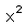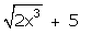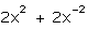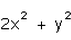# Which of the following is a quadratic polynomial in one variable?a)b)c)d)Correct answer is option 'A'. Can you explain this answer? Related Test: Test: Introduction To Polynomials

## Class 9 QuestionPreet Jun 08, 2018
(a)x^2 is d correct answer becoz it is a quadratic polynomial in variable'x'. (b) is not the correct answer becoz it is not a polynomial becoz it's variable has not an integral power.. it is a rational no. and u know in polynomial he power of variable is always a positive integer. (c)is not the correct answer becoz the power of variable seems negative integer (d)is also an incorrect answer becoz there are two variables x & y.. so (a) is the correct answer. .Arvi Singh Oberoi Dec 26, 2018
Because a quadratic polynomial said that the maximum power as well as it's degree is two...mand in option number 3 we see that it's degree is 2 but after it's other degree is also 2 ...Nandini Kandoi Dec 26, 2018
A is the correct answer bcoz in a quadratic polynomial the highest degree is 2 and so in option A

This discussion on Which of the following is a quadratic polynomial in one variable?a)b)c)d)Correct answer is option 'A'. Can you explain this answer? is done on EduRev Study Group by Class 9 Students. The Questions and Answers of Which of the following is a quadratic polynomial in one variable?a)b)c)d)Correct answer is option 'A'. Can you explain this answer? are solved by group of students and teacher of Class 9, which is also the largest student community of Class 9. If the answer is not available please wait for a while and a community member will probably answer this soon. You can study other questions, MCQs, videos and tests for Class 9 on EduRev and even discuss your questions like Which of the following is a quadratic polynomial in one variable?a)b)c)d)Correct answer is option 'A'. Can you explain this answer? over here on EduRev! Apart from being the largest Class 9 community, EduRev has the largest solved Question bank for Class 9.